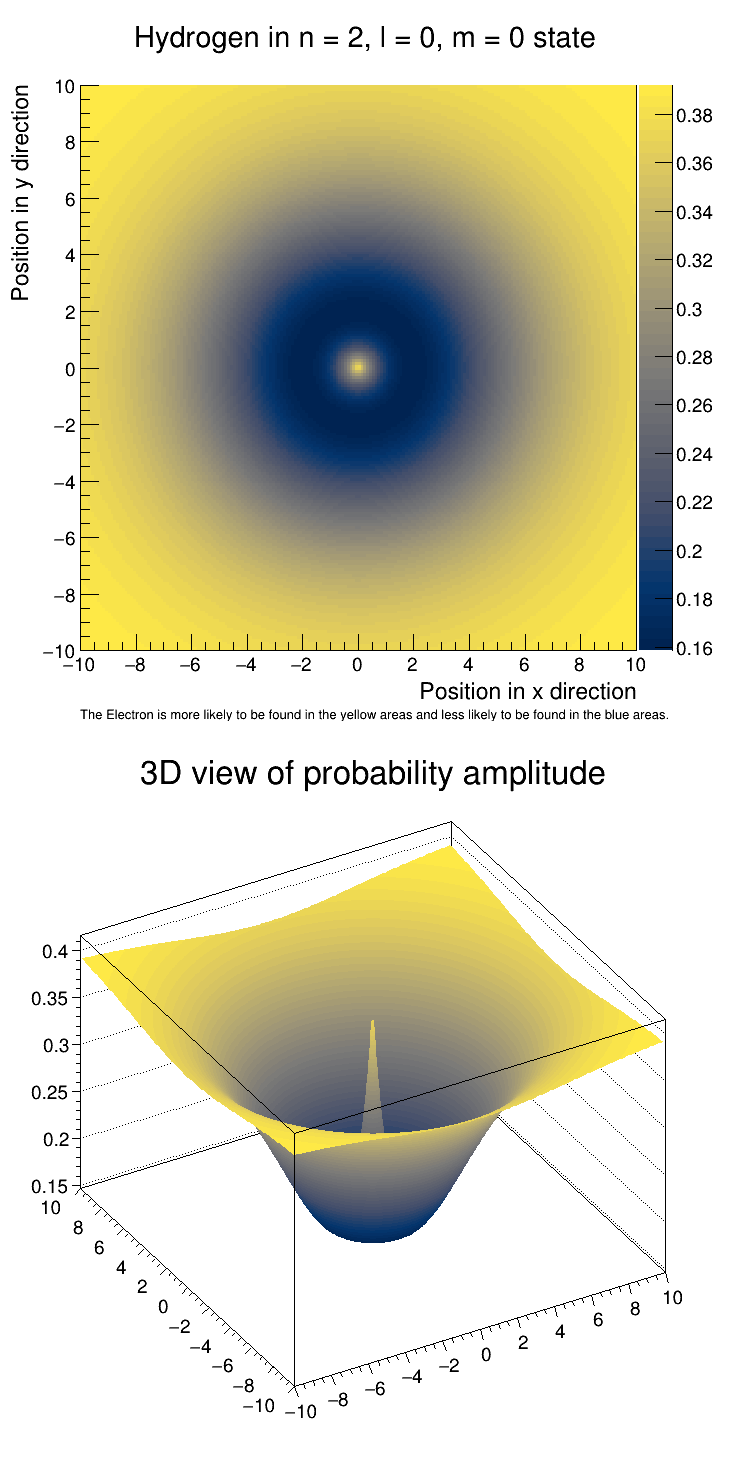# Schroedinger_Hydrogen¶

Plot the Amplitude of a Hydrogen Atom.

Visualize the Amplitude of a Hydrogen Atom in the n = 2, l = 0, m = 0 state. Demonstrates how TH2F can be used in Quantum Mechanics.

The formula for Hydrogen in this energy state is $\psi_{200} = \frac{1}{4\sqrt{2\pi}a_0 ^{\frac{3}{2}}}(2-\frac{\sqrt{x^2+y^2}}{a_0})e^{-\frac{\sqrt{x^2+y^2}}{2a_0}}$

This notebook tutorial was automatically generated with ROOTBOOK-izer from the macro found in the ROOT repository on Monday, September 20, 2021 at 12:55 PM.

In :
%%cpp -d
#include <cmath>


A helper function is created:

In :
%%cpp -d
double WaveFunction(double x, double y) {
double r = sqrt(x *x + y*y);

double w = (1/pow((4*sqrt(2*TMath::Pi())* 1), 1.5)) * (2 - (r / 1)*pow(TMath::E(), (-1 * r)/2)); // Wavefunction formula for psi 2,0,0

return w*w; // Amplitude

}

In :
TH2F *h2D = new TH2F("Hydrogen Atom",
"Hydrogen in n = 2, l = 0, m = 0 state; Position in x direction; Position in y direction",
200, -10, 10, 200, -10, 10);

for (float i = -10; i < 10; i += 0.01) {
for (float j = -10; j < 10; j += 0.01) {
h2D->Fill(i, j, WaveFunction(i, j));
}
}

gStyle->SetPalette(kCividis);
gStyle->SetOptStat(0);

TCanvas *c1 = new TCanvas("c1", "Schroedinger's Hydrogen Atom", 750, 1500);
c1->Divide(1, 2);

auto c1_1 = c1->cd(1);
c1_1->SetRightMargin(0.14);
h2D->GetXaxis()->SetLabelSize(0.03);
h2D->GetYaxis()->SetLabelSize(0.03);
h2D->GetZaxis()->SetLabelSize(0.03);
h2D->SetContour(50);
h2D->Draw("colz");

TLatex *l = new TLatex(-10, -12.43, "The Electron is more likely to be found in the yellow areas and less likely to be found in the blue areas.");
l->SetTextFont(42);
l->SetTextSize(0.02);
l->Draw();

auto c1_2 = c1->cd(2);
c1_2->SetTheta(42.);

TH2D *h2Dc = (TH2D*)h2D->Clone();
h2Dc->SetTitle("3D view of probability amplitude;;");
h2Dc->Draw("surf2");


Draw all canvases

In :
gROOT->GetListOfCanvases()->Draw()